### Multiplication table mazes

Fun to learn the multiplication table! Mazes for learning the multiplication table are fun worksheets that aim to teach the multiplication table using mazes, each of which deals with multiples of numbers, from 2 to 10. In each maze you have to find the way from the beginning to the end, in its multiplication path in 1 to its multiplication in 10. Each worksheet has a bonus: coloring the decorated number. To print the maze page click on it and then save to your computer or print immediately by Ctrl + P.
PS- To save paper and help our planet, you can print on used pages, on the blank side ♥Multiplication maze 2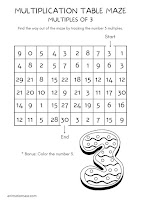Multiplication maze 3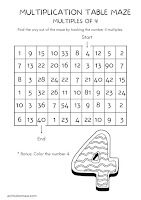Multiplication maze 4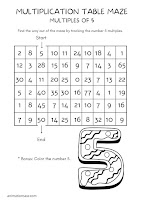Multiplication maze 5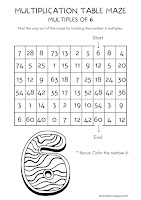Multiplication maze 6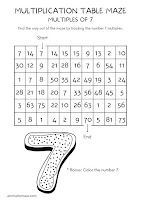Multiplication maze 7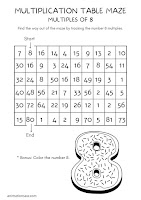Multiplication maze 8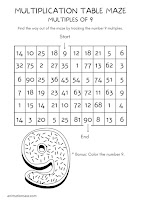Multiplication maze 9Multiplication maze 10